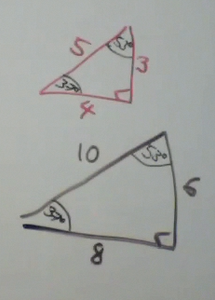## Introduction to Vectors

We have previously mentioned the difference between a scalar and a vector.  A scalar has only a size or magnitude, such as temperature, energy, distance, or speed.

A vector has direction and magnitude.  Examples of vectors are forces, momentum, acceleration, velocity, and displacement.

A vector is drawn like an arrow, with a head and a tail.  The head indicates the direction of the vector.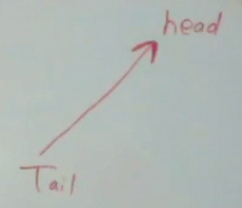We draw the length of the vector  proportional to the magnitude of the vector.  So the longer the vector, the higher the magnitude.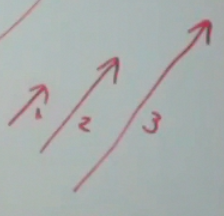We draw the direction of the vector in the appropriate direction such as up or down.Vectors add head to tail.  Suppose you are swimming in a river at a speed of  2 m/s, and the rate of water flow is 3 m/s.  The head of the black vector meets the tail of the green vector.  The resultant vector (result of adding two vectors) is drawn from the tail of the initial vector to the head of the second vector. In this case, 2 + 3 = 5 m/s.  These vectors are colinear or in the same line.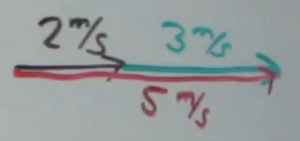However, suppose you are swimming against the river.  You are swimming against the river which is still at 3 m/s.   So the vector starts at the tail of the black vector and finishes again at the head of the green vector.  Here we see the resultant is -1 m/s. These vectors are also colinear.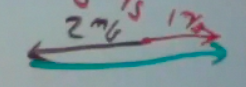Let us suppose an airplane is flying at a speed of 150 knots.  The wind speed is at 50 knots and is in the same direction.  The resultant vector is 200 knots.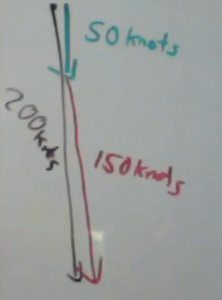Suppose the vectors are not colinear, and they are perpendicular.  In this case, to find the resultant vector, we would have to use the Pythagorean theorem.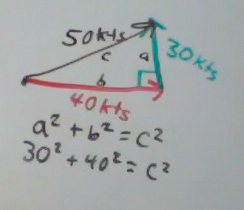We will also be looking at similar triangles.  If you look at the small red triangles with sides a-b-c.  The angle on the left is theta or θ.  The complementary angle to θ would be Φ or phi.  Since we are looking at  a 90° angle, all of the angles add up to 180°.

The large black triangle has sides of A-B-C.  It has the same exact angles, 90, θ, and Φ.

When two triangles have equal angles, they are said to be similar.  The ration of the lengths of the sides are the same, so we say the sides are proportional.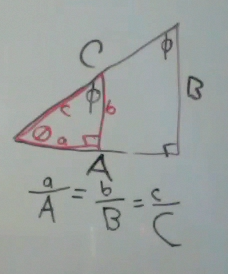A particular easy triangle to understand is the 45°-45°-90° triangle.  If the sides of the triangle have a length of 1, then using the Pythagorean theorem, we find the long side of the triangle (also known as the hypotenuse) has a length of √2.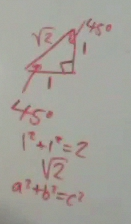Any larger 45° triangle will have sides that are proportionally longer.  If the sides have a length of 2, then using the Pythagorean theorem the hypotenuse is √8.   What we find is that √8 is twice as long as √2.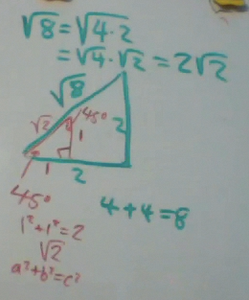Likewise, with a 45° triangle with sides of length 3, the hypotenuse will be 3 times as long.  And 3√2 = √18.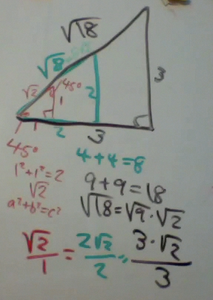Another easy to remember triangle is the  30-60-90 triangle.  If the hypotenuse has a length of 1, then the short side of the triangle of  ½.  This is also the side of the triangle which is opposite of the 30° angle.   The longer side has a length of ½*√3.  This is the side adjacent to the 30° angle.

.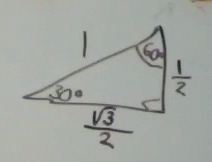If we were to double the length of each side of this 30-60-90 triangle, we have the following triangle.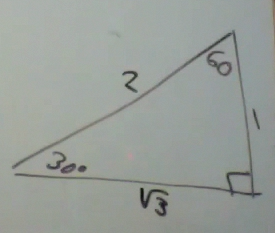Now let us suppose we have a 30-60-90 triangle were the short side has a length of 8.  What would be the length of the other two sides? Using proportionality, we can multiple each side of the above triangle by 8.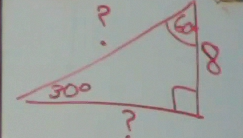Multiplying each side by 8 results in the below triangle.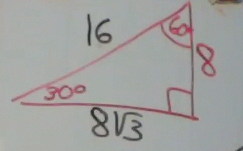The last triangle we will look at is the 3-4-5 triangle.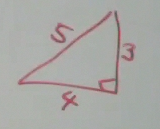If we double each side of this triangle we wind up with….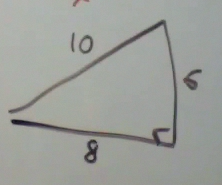All 3-4-5 triangles have equal angles.  The small angle in the triangle is approximately equal to 37°.  The larger angle is approximately equal to 53°.